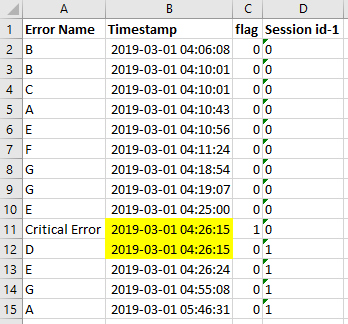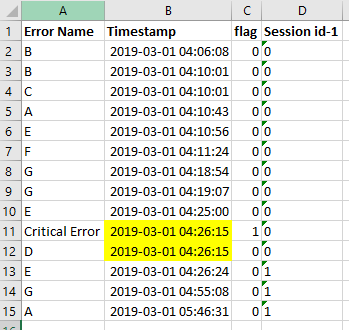# Group examples together in a loop

Member Posts: 9Contributor II
Hello lovely community,

I am currently stuck with a problem of my data prep in RM and I am a bit in a hurry so please excuse my possible spelling mistakes. Here is my problem:In the screenshot you can see a snippet of my data so far. I am working on a way to put my examples into a group of examples, where the last example of a group should be the one with the "Error Name" = Critical Error. You can see the group selection already in the attribute "session id-1", where the row 2-11 is connected to "session id-1"=0.
My problem now is that sometimes an Error occurred on the same time with an Critical Error, but it was written into the group after that Critical Error (as you can see in the first screenshot). Now I am looking for a way to include this event D, which has the same timestamp like the one of the Critical Error, into my first group.

This should be the result:I thought about of getting the max timestamp of "session id-1" = 0 and compare it to the min timestamp of "session-1" = 1 to see if it the same or not but I have great troubles with the loops of RM.

Greets,
Janito

• Member Posts: 662Unicorn
Solution Accepted
If I get it right you basically want to group by Session-id, and want to have the error on the last line?

If so I suggest to loop through values first, where you use your session-id as filter, and then sort on flag (as 0 seems to be ok and 1 is an error) + date to keep your order but add the ones to the end of the row in case of equal timestamp.

See below quick and dirty code example.

```<?xml version="1.0" encoding="UTF-8"?><process version="9.3.001">
<context>
<input/>
<output/>
<macros/>
</context>
<operator activated="true" class="process" compatibility="9.3.001" expanded="true" name="Process">
<parameter key="logverbosity" value="init"/>
<parameter key="random_seed" value="2001"/>
<parameter key="send_mail" value="never"/>
<parameter key="process_duration_for_mail" value="30"/>
<parameter key="encoding" value="UTF-8"/>
<process expanded="true">
<operator activated="true" class="utility:create_exampleset" compatibility="9.3.001" expanded="true" height="68" name="Create ExampleSet" width="90" x="45" y="34">
<parameter key="generator_type" value="comma separated text"/>
<parameter key="number_of_examples" value="100"/>
<parameter key="use_stepsize" value="false"/>
<list key="function_descriptions"/>
<list key="numeric_series_configuration"/>
<list key="date_series_configuration"/>
<list key="date_series_configuration (interval)"/>
<parameter key="date_format" value="yyyy-MM-dd HH:mm:ss"/>
<parameter key="time_zone" value="SYSTEM"/>
<parameter key="input_csv_text" value="Error name&#9;Timestamp&#9;flag&#9;Session id-1&#10;B&#9;09/03/2019 00:00:01&#9;0&#9;0&#10;B&#9;09/03/2019 00:00:02&#9;0&#9;0&#10;C&#9;09/03/2019 00:00:02&#9;0&#9;0&#10;A&#9;09/03/2019 00:00:03&#9;0&#9;0&#10;E&#9;09/03/2019 00:00:04&#9;0&#9;0&#10;F&#9;09/03/2019 00:00:05&#9;0&#9;0&#10;G&#9;09/03/2019 00:00:061&#9;0&#9;0&#10;G&#9;09/03/2019 00:00:07&#9;0&#9;0&#10;E&#9;09/03/2019 00:00:08&#9;0&#9;0&#10;Critical Error&#9;09/03/2019 00:00:09&#9;1&#9;0&#10;D&#9;09/03/2019 00:00:09&#9;0&#9;0&#10;E&#9;09/03/2019 00:00:10&#9;0&#9;1&#10;G&#9;09/03/2019 00:00:11&#9;0&#9;1&#10;A&#9;09/03/2019 00:00:12&#9;0&#9;1&#10;"/>
<parameter key="column_separator" value="\t"/>
<parameter key="parse_all_as_nominal" value="false"/>
<parameter key="decimal_point_character" value="."/>
<parameter key="trim_attribute_names" value="true"/>
</operator>
<operator activated="true" class="numerical_to_polynominal" compatibility="9.3.001" expanded="true" height="82" name="Numerical to Polynominal" width="90" x="179" y="34">
<parameter key="attribute_filter_type" value="single"/>
<parameter key="attribute" value="Session id-1"/>
<parameter key="attributes" value=""/>
<parameter key="use_except_expression" value="false"/>
<parameter key="value_type" value="numeric"/>
<parameter key="use_value_type_exception" value="false"/>
<parameter key="except_value_type" value="real"/>
<parameter key="block_type" value="value_series"/>
<parameter key="use_block_type_exception" value="false"/>
<parameter key="except_block_type" value="value_series_end"/>
<parameter key="invert_selection" value="false"/>
<parameter key="include_special_attributes" value="false"/>
</operator>
<operator activated="true" class="concurrency:loop_values" compatibility="9.3.001" expanded="true" height="82" name="Loop Values" width="90" x="313" y="34">
<parameter key="attribute" value="Session id-1"/>
<parameter key="iteration_macro" value="se"/>
<parameter key="reuse_results" value="false"/>
<parameter key="enable_parallel_execution" value="true"/>
<process expanded="true">
<operator activated="true" class="filter_examples" compatibility="9.3.001" expanded="true" height="103" name="Filter Examples" width="90" x="112" y="34">
<parameter key="parameter_expression" value=""/>
<parameter key="condition_class" value="custom_filters"/>
<parameter key="invert_filter" value="false"/>
<list key="filters_list">
<parameter key="filters_entry_key" value="Session id-1.equals.%{se}"/>
</list>
<parameter key="filters_logic_and" value="true"/>
</operator>
<operator activated="true" class="sort" compatibility="9.3.001" expanded="true" height="82" name="Sort" width="90" x="246" y="34">
<parameter key="attribute_name" value="flag"/>
<parameter key="sorting_direction" value="increasing"/>
</operator>
<operator activated="true" class="sort" compatibility="9.3.001" expanded="true" height="82" name="Sort (2)" width="90" x="380" y="34">
<parameter key="attribute_name" value="Timestamp"/>
<parameter key="sorting_direction" value="increasing"/>
</operator>
<connect from_port="input 1" to_op="Filter Examples" to_port="example set input"/>
<connect from_op="Filter Examples" from_port="example set output" to_op="Sort" to_port="example set input"/>
<connect from_op="Sort" from_port="example set output" to_op="Sort (2)" to_port="example set input"/>
<connect from_op="Sort (2)" from_port="example set output" to_port="output 1"/>
<portSpacing port="source_input 1" spacing="0"/>
<portSpacing port="source_input 2" spacing="0"/>
<portSpacing port="sink_output 1" spacing="0"/>
<portSpacing port="sink_output 2" spacing="0"/>
</process>
</operator>
<operator activated="true" class="append" compatibility="9.3.001" expanded="true" height="82" name="Append" width="90" x="447" y="34">
<parameter key="datamanagement" value="double_array"/>
<parameter key="data_management" value="auto"/>
<parameter key="merge_type" value="all"/>
</operator>
<connect from_op="Create ExampleSet" from_port="output" to_op="Numerical to Polynominal" to_port="example set input"/>
<connect from_op="Numerical to Polynominal" from_port="example set output" to_op="Loop Values" to_port="input 1"/>
<connect from_op="Loop Values" from_port="output 1" to_op="Append" to_port="example set 1"/>
<connect from_op="Append" from_port="merged set" to_port="result 1"/>
<portSpacing port="source_input 1" spacing="0"/>
<portSpacing port="sink_result 1" spacing="0"/>
<portSpacing port="sink_result 2" spacing="0"/>
</process>
</operator>
</process>
```

• Member Posts: 9Contributor II
edited July 2019
Edit @ kayman:
I changed the lag after sorting first for the flag and then for the timestamp and it worked perfectly!
Thank you so much, you saved my day!Hey Kayman,

thanks a lot, your workflow works perfectly for me but my input data is not the one of picture 2, instead its from picture 1.
Attached you will find the wf with the create table set.

`<?xml version="1.0" encoding="UTF-8"?><process version="9.2.001"><br>&nbsp; <context><br>&nbsp;&nbsp;&nbsp; <input/><br>&nbsp;&nbsp;&nbsp; <output/><br>&nbsp;&nbsp;&nbsp; <macros/><br>&nbsp; </context><br>&nbsp; <operator activated="true" class="process" compatibility="9.2.001" expanded="true" name="Process"><br>&nbsp;&nbsp;&nbsp; <parameter key="logverbosity" value="init"/><br>&nbsp;&nbsp;&nbsp; <parameter key="random_seed" value="2001"/><br>&nbsp;&nbsp;&nbsp; <parameter key="send_mail" value="never"/><br>&nbsp;&nbsp;&nbsp; <parameter key="notification_email" value=""/><br>&nbsp;&nbsp;&nbsp; <parameter key="process_duration_for_mail" value="30"/><br>&nbsp;&nbsp;&nbsp; <parameter key="encoding" value="UTF-8"/><br>&nbsp;&nbsp;&nbsp; <process expanded="true"><br>&nbsp;&nbsp;&nbsp;&nbsp;&nbsp; <operator activated="true" breakpoints="after" class="utility:create_exampleset" compatibility="9.2.001" expanded="true" height="68" name="Create ExampleSet" width="90" x="45" y="34"><br>&nbsp;&nbsp;&nbsp;&nbsp;&nbsp;&nbsp;&nbsp; <parameter key="generator_type" value="comma separated text"/><br>&nbsp;&nbsp;&nbsp;&nbsp;&nbsp;&nbsp;&nbsp; <parameter key="number_of_examples" value="100"/><br>&nbsp;&nbsp;&nbsp;&nbsp;&nbsp;&nbsp;&nbsp; <parameter key="use_stepsize" value="false"/><br>&nbsp;&nbsp;&nbsp;&nbsp;&nbsp;&nbsp;&nbsp; <list key="function_descriptions"/><br>&nbsp;&nbsp;&nbsp;&nbsp;&nbsp;&nbsp;&nbsp; <parameter key="add_id_attribute" value="false"/><br>&nbsp;&nbsp;&nbsp;&nbsp;&nbsp;&nbsp;&nbsp; <list key="numeric_series_configuration"/><br>&nbsp;&nbsp;&nbsp;&nbsp;&nbsp;&nbsp;&nbsp; <list key="date_series_configuration"/><br>&nbsp;&nbsp;&nbsp;&nbsp;&nbsp;&nbsp;&nbsp; <list key="date_series_configuration (interval)"/><br>&nbsp;&nbsp;&nbsp;&nbsp;&nbsp;&nbsp;&nbsp; <parameter key="date_format" value="yyyy-MM-dd HH:mm:ss"/><br>&nbsp;&nbsp;&nbsp;&nbsp;&nbsp;&nbsp;&nbsp; <parameter key="time_zone" value="SYSTEM"/><br>&nbsp;&nbsp;&nbsp;&nbsp;&nbsp;&nbsp;&nbsp; <parameter key="input_csv_text" value="Error name&#9;Timestamp&#9;flag&#9;Session id-1&#10;B&#9;09/03/2019 00:00:01&#9;0&#9;0&#10;B&#9;09/03/2019 00:00:02&#9;0&#9;0&#10;C&#9;09/03/2019 00:00:02&#9;0&#9;0&#10;A&#9;09/03/2019 00:00:03&#9;0&#9;0&#10;E&#9;09/03/2019 00:00:04&#9;0&#9;0&#10;F&#9;09/03/2019 00:00:05&#9;0&#9;0&#10;G&#9;09/03/2019 00:00:061&#9;0&#9;0&#10;G&#9;09/03/2019 00:00:07&#9;0&#9;0&#10;E&#9;09/03/2019 00:00:08&#9;0&#9;0&#10;Critical Error&#9;09/03/2019 00:00:09&#9;1&#9;0&#10;D&#9;09/03/2019 00:00:09&#9;0&#9;1&#10;E&#9;09/03/2019 00:00:10&#9;0&#9;1&#10;G&#9;09/03/2019 00:00:11&#9;0&#9;1&#10;A&#9;09/03/2019 00:00:12&#9;0&#9;1&#10;"/><br>&nbsp;&nbsp;&nbsp;&nbsp;&nbsp;&nbsp;&nbsp; <parameter key="column_separator" value="\t"/><br>&nbsp;&nbsp;&nbsp;&nbsp;&nbsp;&nbsp;&nbsp; <parameter key="parse_all_as_nominal" value="false"/><br>&nbsp;&nbsp;&nbsp;&nbsp;&nbsp;&nbsp;&nbsp; <parameter key="decimal_point_character" value="."/><br>&nbsp;&nbsp;&nbsp;&nbsp;&nbsp;&nbsp;&nbsp; <parameter key="trim_attribute_names" value="true"/><br>&nbsp;&nbsp;&nbsp;&nbsp;&nbsp; </operator><br>&nbsp;&nbsp;&nbsp;&nbsp;&nbsp; <connect from_op="Create ExampleSet" from_port="output" to_port="result 1"/><br>&nbsp;&nbsp;&nbsp;&nbsp;&nbsp; <portSpacing port="source_input 1" spacing="0"/><br>&nbsp;&nbsp;&nbsp;&nbsp;&nbsp; <portSpacing port="sink_result 1" spacing="0"/><br>&nbsp;&nbsp;&nbsp;&nbsp;&nbsp; <portSpacing port="sink_result 2" spacing="0"/><br>&nbsp;&nbsp;&nbsp; </process><br>&nbsp; </operator><br></process>`
The flag indicates an Critical Error found in the example so my groups will be formed until the flag of value "1" is reached. But sometimes an event with the same timestamp was written after the Critical Error into the table. Maybe it could be possible to sort the whole table in the beginning for the timestamps? But in this case the last value have to be the Critical Error for all cases.

Greets
Janito
• Moderator, Employee, RMResearcher, Member Posts: 297RM Data Scientist
Hi @Janito ,
You could create a new Attribute based on the date and the Error Attribute. Then you can sort by this Attribute.
1. Copy Date and Error Attribute
2. Convert date to nominal Attribute (e.g. in Format yyyy-MM-dd HH:mm:ss)
3. Prepend something like "111_" where Error = "Critical Error" (e.g. using Replace)
4. Concatenate Date and Error (in this order)
5. Sort Ascending
You can also do steps 1-4 within one Generate Attributes Operator.

Happy Mining,
Edin
• Member Posts: 3Contributor I
excellent work